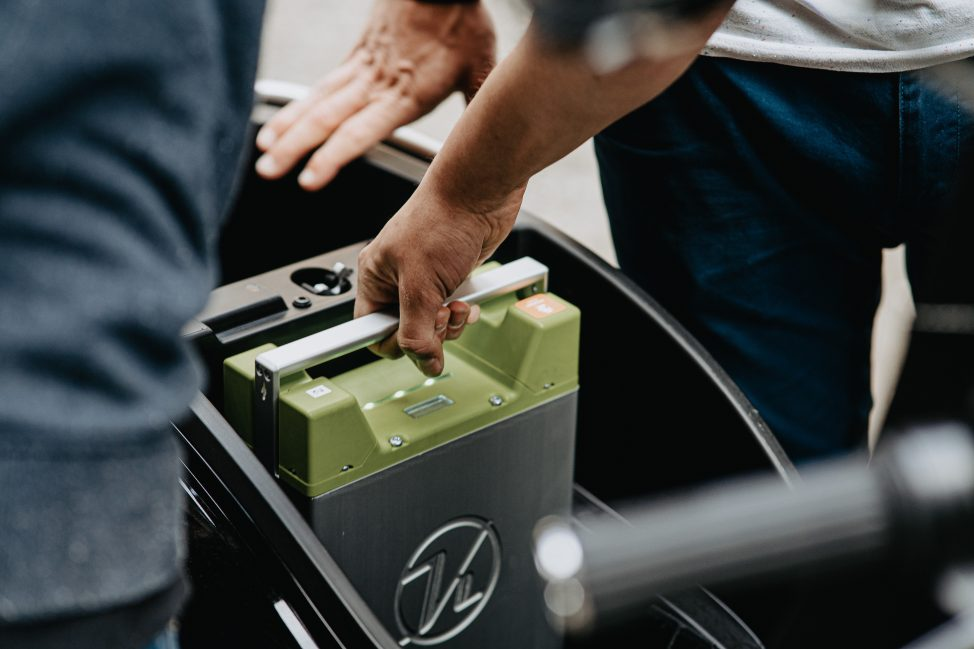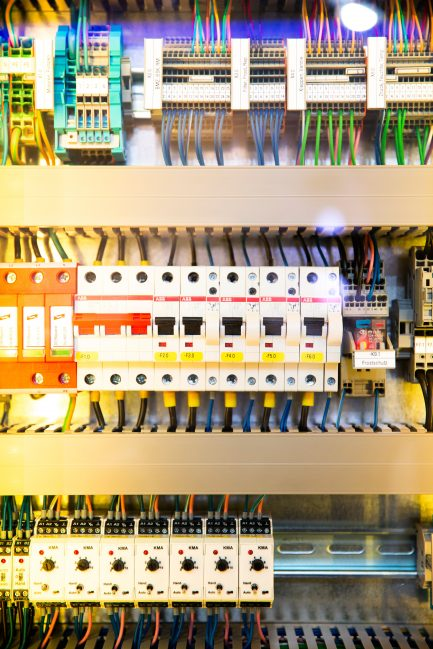# How Fast Will a Power Inverter Drain a Battery?

In the not-too-distant past, power inverters were notorious for draining entire batteries before you knew it. However, due to significant advancements in materials and engineering, power inverters have become more efficient than ever before. This then raises the question, how long does it take for a battery to completely discharge and drain fully while connected to an inverter. As we will see, this depends on a number of factors and varies with different use cases.

## How Fast Will a Power Inverter Drain a Standard Battery?First of all, let us start our investigation with a simple review of how inverters work. An inverter is a device that converts a direct current (DC) into an alternating current (AC). Typically, most devices we plug in to our homes rely on AC current, however, when we wish to use a battery or solar panel, we need a safe and efficient way to convert the DC current to AC.

### Reviewing How Power Inverters Work

A typical inverter comes with a simple Watt rating. A watt is just a unit for power, which as an aside, tells you the energy consumption of a device per second. Note that the Watt rating of an inverter does not tell you the amount of power drawn from whatever load you are using. It simply tells you the maximum load that the inverter can support. Watt ratings can vary between 50 watts to 50,000 watts, although the latter power rating only applies to industrial uses.

### Amp Hours vs. Watt Hours

Another common confusion for many people is the difference between amp hour (Ah) and watt hours (Wh). A watt hour is a unit of energy, it tells you the total amount of energy consumed by a device in a given amount of time. For example, if you run a 100 W light bulb for 4 hours, it consumes 400 Wh of energy and you will be charged that by your utility provider.

An amp hour, on the other hand, is a typical measure for battery capacity and tells you how much charge a battery can hold. To actually find the complete energy capacity of a battery, we need to multiply the battery capacity in amp hours by the voltage the battery is running at.

So for example, a regular gas car battery typically has a 100 Ah battery capacity with a 12 V voltage for a total energy capacity of 1200 Wh or 1.2 kWh (kWh = 1000 Wh). A typical electric vehicle has only around a 200 Ah battery capacity, but runs at a whopping 350 V for a total energy capacity of 70 kWh. As we can clearly see, the amp hours of a battery are just one component of the total energy of a battery.

### Determining How Long a Battery will Last When Using a Power InverterThere are a number of other factors that help determine the amount of time a battery lasts when attached to an inverter. The inverter efficiency is a measure of how much power is lost when we convert DC current into AC current. Most modern inverters have very high efficiencies, often approaching 90%+. Pure sine wave inverters tend to be more expensive, but are almost always more efficient than modified sine wave inverters.

#### Inverter Size

Now, we have to account for the inverter size, which in this case just means the power rating of an inverter. Usually, an inverter has two different ratings: continuous power and peak power. The continuous power is more important if you want to calculate the total length of time for an inverter system, while the peak power rating is important for safety and reliability reasons (if you want to run a heavy duty refrigeration system, you definitely want to pay attention to the peak power rating).

Lastly, the load size is also important to account for when trying to calculate the total amount of time a battery will last for when connected to an inverter. The load size will almost always be printed on the device you are trying to run. For example, a light bulb might consume 50 Watts, a typical TV will consume 300 Watts and a heater will consume 1000 Watts. Before you run your inverter, always check to see the total amount of watts connected to it.

### Time to do the Math

Alright, let’s do a simple example to calculate the amount of time a battery will last when connected to an inverter. Say that we have a battery of 12 V with a capacity of 100 Ah and we are running an inverter that is 90% efficient with a 300 W load (say a TV). How long does it take to completely discharge the battery?

1. Let’s begin by calculating the total energy capacity of the battery which is simply 12 x 100 = 1200 Wh.
2. The load is 300 W. Because of the inverter’s inefficiency and to deal with the initial load current, a good rule of thumb is to add 20% to the power consumption of the given load. In this case, this means that the total approximate load is 300 + 300 x 0.20 = 360 W.
3. In order to find the time that the TV will stay on, we divide the total power capacity of the battery by our estimated load which in this case means a length of 1200 / 360 = 3.3 hours.

Note that this is an approximation! In reality, to get the exact amount of time a battery will last, we need to account for voltage drops in the battery, performance of the TV, etc. so it usually makes sense to take this calculated value as the absolute maximum amount of time you can run your device. Indeed, it is usually actively harmful to discharge your battery completely to zero and you should try to remember not to drain it all the way.

## Conclusion

In conclusion, the most important thing to know when trying to approximate the amount of time your devices will run off an inverter is the energy capacity of your battery and the total power consumption of your load. It is also important to make sure to get an inverter that is above your total expected power load.